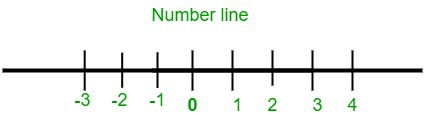# What are negative numbers?

• Last Updated : 10 Dec, 2021

Algebra is a standard subject of mathematics that is about the study of the evaluation of numbers and symbols. The algebraic operations are carried out to determine the unknown values represented by symbols or alphabets in the expression. Algebraic equations are associated with terms like a variable, constants, factors, and coefficient of variables.

### Number line

A number line is a mathematical representation of numbers on a straight line placed at equal intervals. There are three types of numbers represented in a numbers line. These numbers are positive numbers, zero, and negative numbers.

In a number line, the zero lies at the center which acts as a neutral value. It’s neither negative nor positive. While moving towards the left from zero the values start to decrease and these values are known as negative numbers. Whereas moving towards the right from zero the values start to increase and these values are known as positive numbers.

1. Number without a minus sign is a positive number.
2. Number with a minus sign is a negative number.### What are negative numbers?

Negative numbers are basically defined as the numbers which are less than zero. Negative numbers are placed on the left side of the number line from zero. As we keep moving towards the left from zero in the number line the value of numbers decreases. These numbers are signified by the sign minus (-) which determines the value as negative.

Negative numbers can also be understood as the opposite value of positive numbers. The set of negative numbers counts from -1, -2, -3, -4, -5, … and so on.

### Sample problems

Question 1: Can the symbols used in algebra be negative?

Yes, the symbols used in algebra with negative signs are considered negative.

Question 2: If a number does not have any sign attached to it, which type of number it is?

If a number has no sign attached to it, the number is said to be a positive number.

Question 3: Where are negative numbers used?

Negative numbers are mostly used in weather forecasting to define the temperature or humidity in the environment.

Question 4: What will be the result if a 6 is subtracted by 8?

If 6 is subtracted by 8 the result will be -2 which is a negative number.

6 – 8 = -2

Question 5: What will be the difference between 9 and 6?